Publicité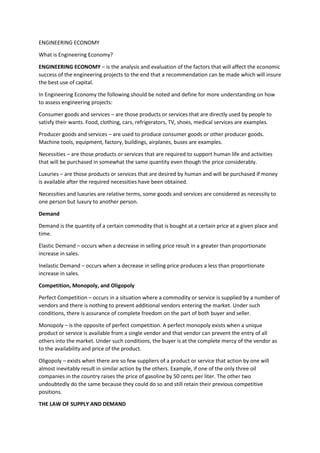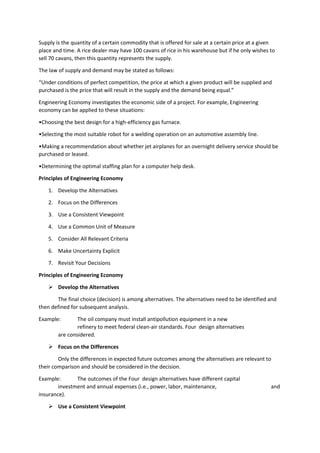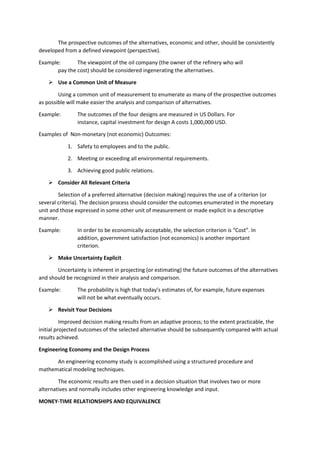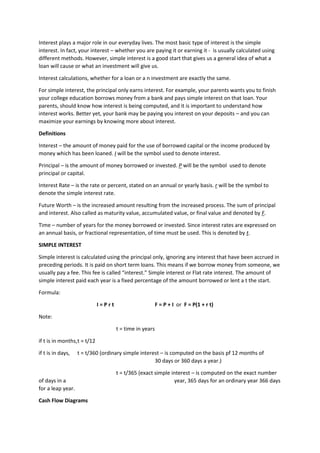Publicité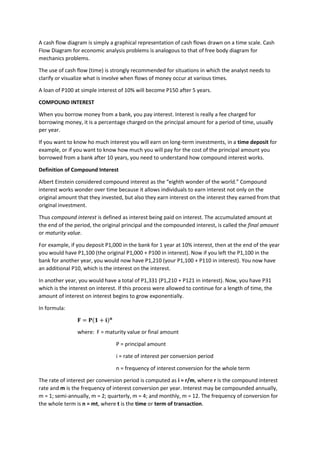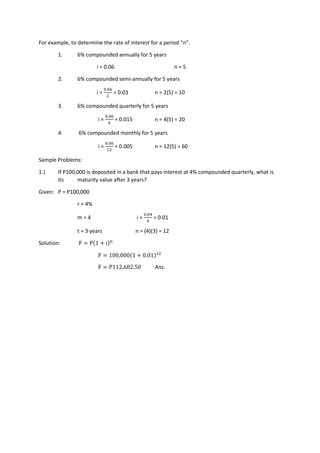Prochain SlideShare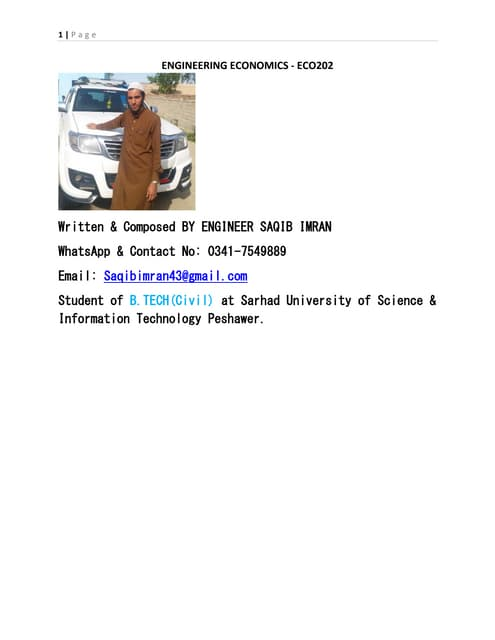Engineering economics ECO-202
Chargement dans ... 3
1 sur 6
Publicité

### ENGINEERING-ECONOMY.docx

1. ENGINEERING ECONOMY What is Engineering Economy? ENGINEERING ECONOMY – is the analysis and evaluation of the factors that will affect the economic success of the engineering projects to the end that a recommendation can be made which will insure the best use of capital. In Engineering Economy the following should be noted and define for more understanding on how to assess engineering projects: Consumer goods and services – are those products or services that are directly used by people to satisfy their wants. Food, clothing, cars, refrigerators, TV, shoes, medical services are examples. Producer goods and services – are used to produce consumer goods or other producer goods. Machine tools, equipment, factory, buildings, airplanes, buses are examples. Necessities – are those products or services that are required to support human life and activities that will be purchased in somewhat the same quantity even though the price considerably. Luxuries – are those products or services that are desired by human and will be purchased if money is available after the required necessities have been obtained. Necessities and luxuries are relative terms, some goods and services are considered as necessity to one person but luxury to another person. Demand Demand is the quantity of a certain commodity that is bought at a certain price at a given place and time. Elastic Demand – occurs when a decrease in selling price result in a greater than proportionate increase in sales. Inelastic Demand – occurs when a decrease in selling price produces a less than proportionate increase in sales. Competition, Monopoly, and Oligopoly Perfect Competition – occurs in a situation where a commodity or service is supplied by a number of vendors and there is nothing to prevent additional vendors entering the market. Under such conditions, there is assurance of complete freedom on the part of both buyer and seller. Monopoly – is the opposite of perfect competition. A perfect monopoly exists when a unique product or service is available from a single vendor and that vendor can prevent the entry of all others into the market. Under such conditions, the buyer is at the complete mercy of the vendor as to the availability and price of the product. Oligopoly – exists when there are so few suppliers of a product or service that action by one will almost inevitably result in similar action by the others. Example, if one of the only three oil companies in the country raises the price of gasoline by 50 cents per liter. The other two undoubtedly do the same because they could do so and still retain their previous competitive positions. THE LAW OF SUPPLY AND DEMAND
2. Supply is the quantity of a certain commodity that is offered for sale at a certain price at a given place and time. A rice dealer may have 100 cavans of rice in his warehouse but if he only wishes to sell 70 cavans, then this quantity represents the supply. The law of supply and demand may be stated as follows: “Under conditions of perfect competition, the price at which a given product will be supplied and purchased is the price that will result in the supply and the demand being equal.” Engineering Economy investigates the economic side of a project. For example, Engineering economy can be applied to these situations: •Choosing the best design for a high-efficiency gas furnace. •Selecting the most suitable robot for a welding operation on an automotive assembly line. •Making a recommendation about whether jet airplanes for an overnight delivery service should be purchased or leased. •Determining the optimal staffing plan for a computer help desk. Principles of Engineering Economy 1. Develop the Alternatives 2. Focus on the Differences 3. Use a Consistent Viewpoint 4. Use a Common Unit of Measure 5. Consider All Relevant Criteria 6. Make Uncertainty Explicit 7. Revisit Your Decisions Principles of Engineering Economy  Develop the Alternatives The final choice (decision) is among alternatives. The alternatives need to be identified and then defined for subsequent analysis. Example: The oil company must install antipollution equipment in a new refinery to meet federal clean-air standards. Four design alternatives are considered.  Focus on the Differences Only the differences in expected future outcomes among the alternatives are relevant to their comparison and should be considered in the decision. Example: The outcomes of the Four design alternatives have different capital investment and annual expenses (i.e., power, labor, maintenance, and insurance).  Use a Consistent Viewpoint
3. The prospective outcomes of the alternatives, economic and other, should be consistently developed from a defined viewpoint (perspective). Example: The viewpoint of the oil company (the owner of the refinery who will pay the cost) should be considered ingenerating the alternatives.  Use a Common Unit of Measure Using a common unit of measurement to enumerate as many of the prospective outcomes as possible will make easier the analysis and comparison of alternatives. Example: The outcomes of the four designs are measured in US Dollars. For instance, capital investment for design A costs 1,000,000 USD. Examples of Non-monetary (not economic) Outcomes: 1. Safety to employees and to the public. 2. Meeting or exceeding all environmental requirements. 3. Achieving good public relations.  Consider All Relevant Criteria Selection of a preferred alternative (decision making) requires the use of a criterion (or several criteria). The decision process should consider the outcomes enumerated in the monetary unit and those expressed in some other unit of measurement or made explicit in a descriptive manner. Example: In order to be economically acceptable, the selection criterion is “Cost”. In addition, government satisfaction (not economics) is another important criterion.  Make Uncertainty Explicit Uncertainty is inherent in projecting (or estimating) the future outcomes of the alternatives and should be recognized in their analysis and comparison. Example: The probability is high that today’s estimates of, for example, future expenses will not be what eventually occurs.  Revisit Your Decisions Improved decision making results from an adaptive process; to the extent practicable, the initial projected outcomes of the selected alternative should be subsequently compared with actual results achieved. Engineering Economy and the Design Process An engineering economy study is accomplished using a structured procedure and mathematical modeling techniques. The economic results are then used in a decision situation that involves two or more alternatives and normally includes other engineering knowledge and input. MONEY-TIME RELATIONSHIPS AND EQUIVALENCE
4. Interest plays a major role in our everyday lives. The most basic type of interest is the simple interest. In fact, your interest – whether you are paying it or earning it - is usually calculated using different methods. However, simple interest is a good start that gives us a general idea of what a loan will cause or what an investment will give us. Interest calculations, whether for a loan or a n investment are exactly the same. For simple interest, the principal only earns interest. For example, your parents wants you to finish your college education borrows money from a bank and pays simple interest on that loan. Your parents, should know how interest is being computed, and it is important to understand how interest works. Better yet, your bank may be paying you interest on your deposits – and you can maximize your earnings by knowing more about interest. Definitions Interest – the amount of money paid for the use of borrowed capital or the income produced by money which has been loaned. I will be the symbol used to denote interest. Principal – is the amount of money borrowed or invested. P will be the symbol used to denote principal or capital. Interest Rate – is the rate or percent, stated on an annual or yearly basis. r will be the symbol to denote the simple interest rate. Future Worth – is the increased amount resulting from the increased process. The sum of principal and interest. Also called as maturity value, accumulated value, or final value and denoted by F. Time – number of years for the money borrowed or invested. Since interest rates are expressed on an annual basis, or fractional representation, of time must be used. This is denoted by t. SIMPLE INTEREST Simple interest is calculated using the principal only, ignoring any interest that have been accrued in preceding periods. It is paid on short term loans. This means if we borrow money from someone, we usually pay a fee. This fee is called “interest.” Simple interest or Flat rate interest. The amount of simple interest paid each year is a fixed percentage of the amount borrowed or lent a t the start. Formula: I = P r t F = P + I or F = P(1 + r t) Note: t = time in years if t is in months,t = t/12 if t is in days, t = t/360 (ordinary simple interest – is computed on the basis pf 12 months of 30 days or 360 days a year.) t = t/365 (exact simple interest – is computed on the exact number of days in a year, 365 days for an ordinary year 366 days for a leap year. Cash Flow Diagrams
5. A cash flow diagram is simply a graphical representation of cash flows drawn on a time scale. Cash Flow Diagram for economic analysis problems is analogous to that of free body diagram for mechanics problems. The use of cash flow (time) is strongly recommended for situations in which the analyst needs to clarify or visualize what is involve when flows of money occur at various times. A loan of P100 at simple interest of 10% will become P150 after 5 years. COMPOUND INTEREST When you borrow money from a bank, you pay interest. Interest is really a fee charged for borrowing money, it is a percentage charged on the principal amount for a period of time, usually per year. If you want to know ho much interest you will earn on long-term investments, in a time deposit for example, or if you want to know how much you will pay for the cost of the principal amount you borrowed from a bank after 10 years, you need to understand how compound interest works. Definition of Compound Interest Albert Einstein considered compound interest as the “eighth wonder of the world.” Compound interest works wonder over time because it allows individuals to earn interest not only on the original amount that they invested, but also they earn interest on the interest they earned from that original investment. Thus compound interest is defined as interest being paid on interest. The accumulated amount at the end of the period, the original principal and the compounded interest, is called the final amount or maturity value. For example, if you deposit P1,000 in the bank for 1 year at 10% interest, then at the end of the year you would have P1,100 (the original P1,000 + P100 in interest). Now if you left the P1,100 in the bank for another year, you would now have P1,210 (your P1,100 + P110 in interest). You now have an additional P10, which is the interest on the interest. In another year, you would have a total of P1,331 (P1,210 + P121 in interest). Now, you have P31 which is the interest on interest. If this process were allowed to continue for a length of time, the amount of interest on interest begins to grow exponentially. In formula: 𝐅 = 𝐏(𝟏 + 𝐢)𝐧 where: F = maturity value or final amount P = principal amount i = rate of interest per conversion period n = frequency of interest conversion for the whole term The rate of interest per conversion period is computed as i = r/m, where r is the compound interest rate and m is the frequency of interest conversion per year. Interest may be compounded annually, m = 1; semi-annually, m = 2; quarterly, m = 4; and monthly, m = 12. The frequency of conversion for the whole term is n = mt, where t is the time or term of transaction.
6. For example, to determine the rate of interest for a period “n”. 1. 6% compounded annually for 5 years i = 0.06 n = 5 2. 6% compounded semi-annually for 5 years i = 0.06 2 = 0.03 n = 2(5) = 10 3. 6% compounded quarterly for 5 years i = 0.06 4 = 0.015 n = 4(5) = 20 4. 6% compounded monthly for 5 years i = 0.06 12 = 0.005 n = 12(5) = 60 Sample Problems: 1.) If P100,000 is deposited in a bank that pays interest at 4% compounded quarterly, what is its maturity value after 3 years? Given: P = P100,000 r = 4% m = 4 i = 0.04 4 = 0.01 t = 3 years n = (4)(3) = 12 Solution: F = P(1 + i)n F = 100,000(1 + 0.01)12 F = P112,682.50 Ans.
Publicité